# Binary Search CS 5010 Program Design Paradigms Bootcamp

• Slides: 17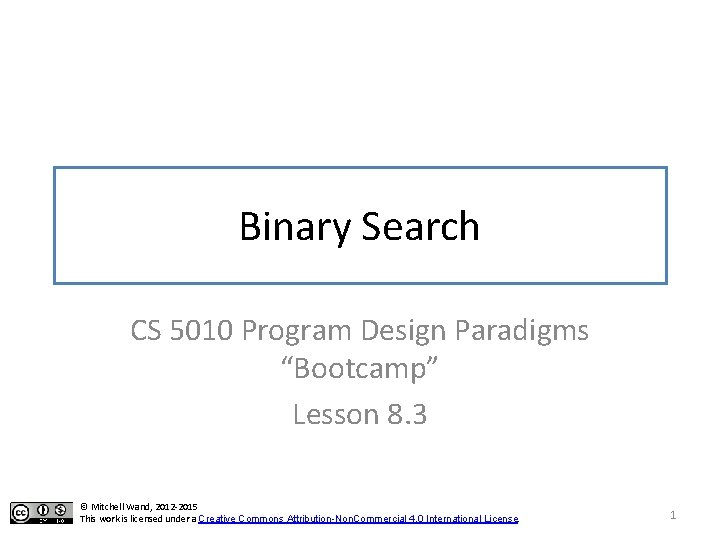Introduction • Binary search is a classic example that illustrates general recursion • We will look at a function for binary search 2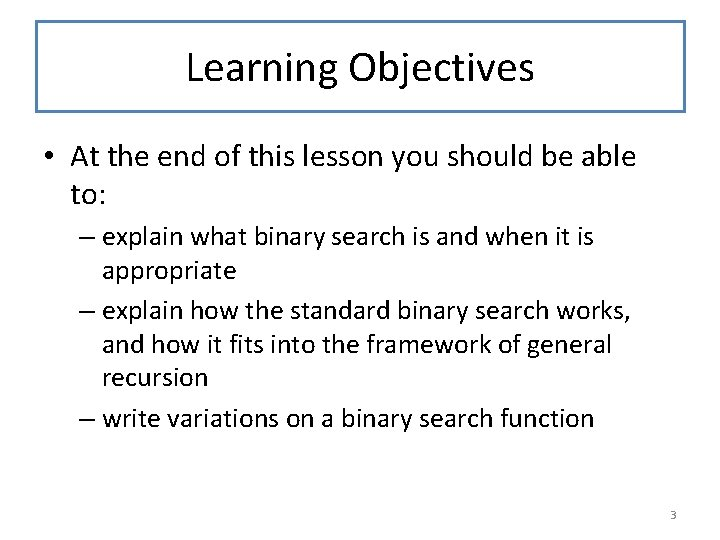Learning Objectives • At the end of this lesson you should be able to: – explain what binary search is and when it is appropriate – explain how the standard binary search works, and how it fits into the framework of general recursion – write variations on a binary search function 3Binary Search • You probably learned about binary search in an array: given an array A[0: N] of increasing values and a target tgt, find an i such that A[i] = tgt, or else report not found. 4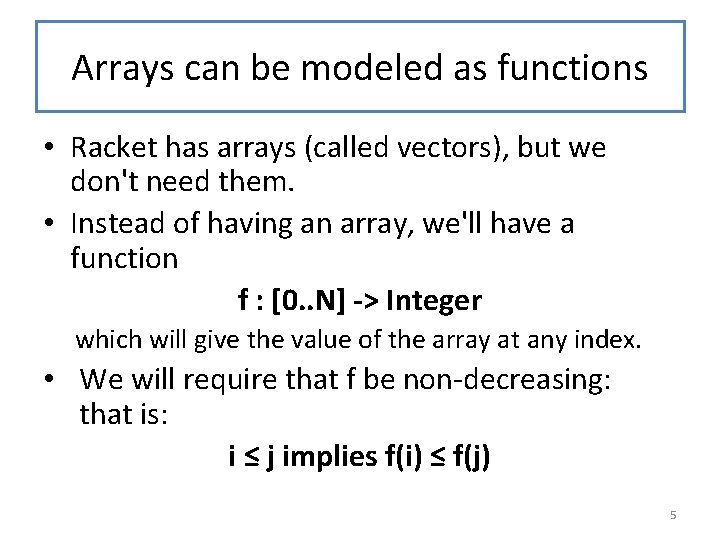Arrays can be modeled as functions • Racket has arrays (called vectors), but we don't need them. • Instead of having an array, we'll have a function f : [0. . N] -> Integer which will give the value of the array at any index. • We will require that f be non-decreasing: that is: i ≤ j implies f(i) ≤ f(j) 5Let's do the obvious generalization • Clearly the 0 and N don't matter, so we'll add them as arguments to our function. 6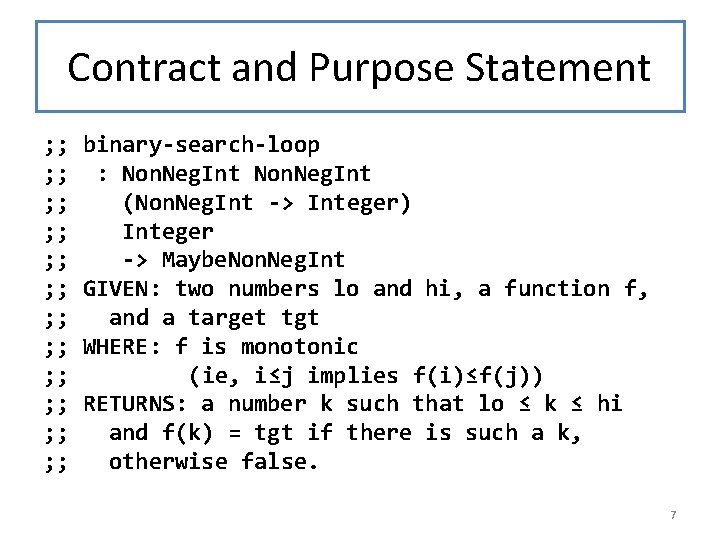Contract and Purpose Statement ; ; ; ; ; ; binary-search-loop : Non. Neg. Int (Non. Neg. Int -> Integer) Integer -> Maybe. Non. Neg. Int GIVEN: two numbers lo and hi, a function f, and a target tgt WHERE: f is monotonic (ie, i≤j implies f(i)≤f(j)) RETURNS: a number k such that lo ≤ k ≤ hi and f(k) = tgt if there is such a k, otherwise false. 7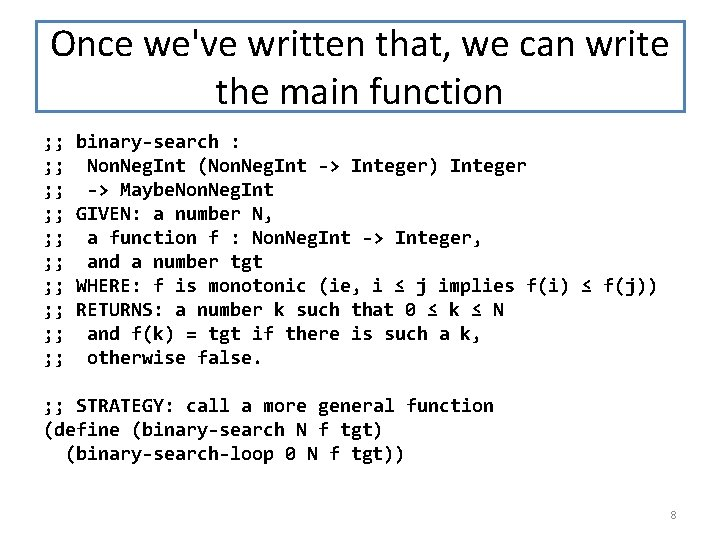Once we've written that, we can write the main function ; ; ; ; ; binary-search : Non. Neg. Int (Non. Neg. Int -> Integer) Integer -> Maybe. Non. Neg. Int GIVEN: a number N, a function f : Non. Neg. Int -> Integer, and a number tgt WHERE: f is monotonic (ie, i ≤ j implies f(i) ≤ f(j)) RETURNS: a number k such that 0 ≤ k ≤ N and f(k) = tgt if there is such a k, otherwise false. ; ; STRATEGY: call a more general function (define (binary-search N f tgt) (binary-search-loop 0 N f tgt)) 8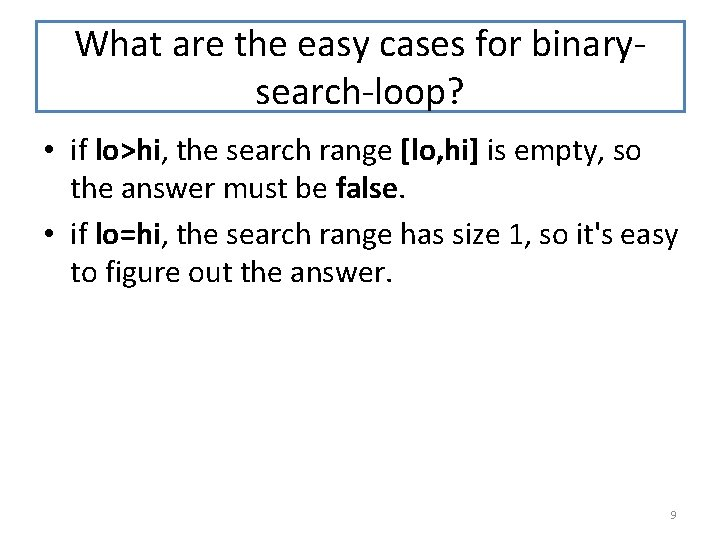What are the easy cases for binarysearch-loop? • if lo>hi, the search range [lo, hi] is empty, so the answer must be false. • if lo=hi, the search range has size 1, so it's easy to figure out the answer. 9What if the search range is larger? • Insight of binary search: divide it in half. • At this point we know that lo < hi. • Choose a midpoint p in [lo, hi]. – p doesn't have to be close to the center– any value in [lo, hi] will lead to a correct program – but choosing p to be near the center means that the search space is divided in half every time, so you'll only need about log₂(hi-lo) steps. 10What are the cases? • f(p) < tgt – so we can rule out p, and all values less than p (because if p' < p, f(p') ≤ f(p) < tgt). – So the answer k, if it exists, is in [p+1, hi] • tgt < f(p) – so we can rule out p and all values greater than p, because if p < p', tgt < f(p) ≤ f(p'). – So the answer k, if it exists, is in [lo, p-1] • tgt = f(p) – then p is our desired k. 11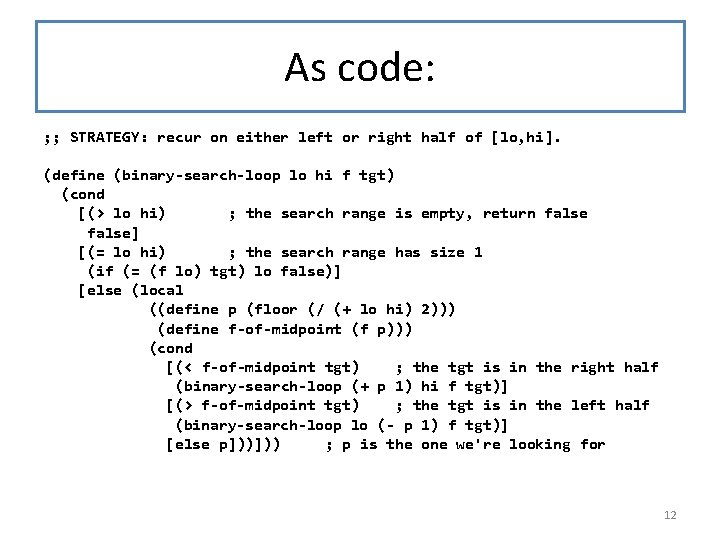As code: ; ; STRATEGY: recur on either left or right half of [lo, hi]. (define (binary-search-loop lo hi f tgt) (cond [(> lo hi) ; the search range is empty, return false] [(= lo hi) ; the search range has size 1 (if (= (f lo) tgt) lo false)] [else (local ((define p (floor (/ (+ lo hi) 2))) (define f-of-midpoint (f p))) (cond [(< f-of-midpoint tgt) ; the tgt is in the right half (binary-search-loop (+ p 1) hi f tgt)] [(> f-of-midpoint tgt) ; the tgt is in the left half (binary-search-loop lo (- p 1) f tgt)] [else p]))])) ; p is the one we're looking for 12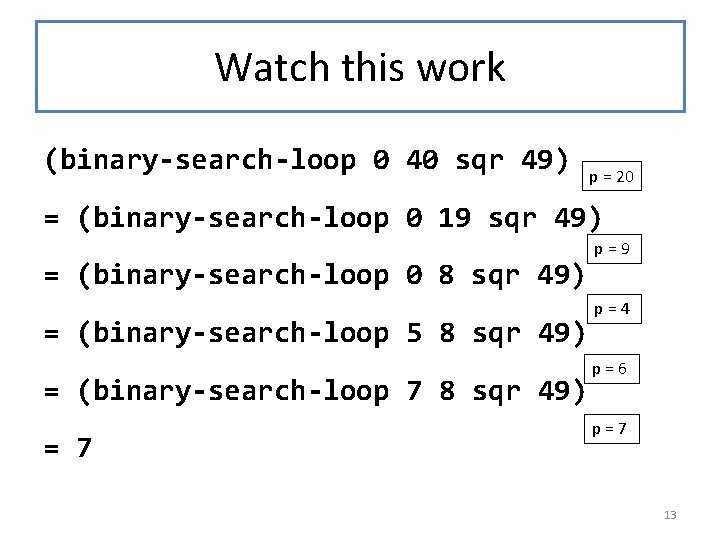Watch this work (binary-search-loop 0 40 sqr 49) p = 20 = (binary-search-loop 0 19 sqr 49) = (binary-search-loop 0 8 sqr 49) = (binary-search-loop 5 8 sqr 49) = (binary-search-loop 7 8 sqr 49) = 7 p=9 p=4 p=6 p=7 13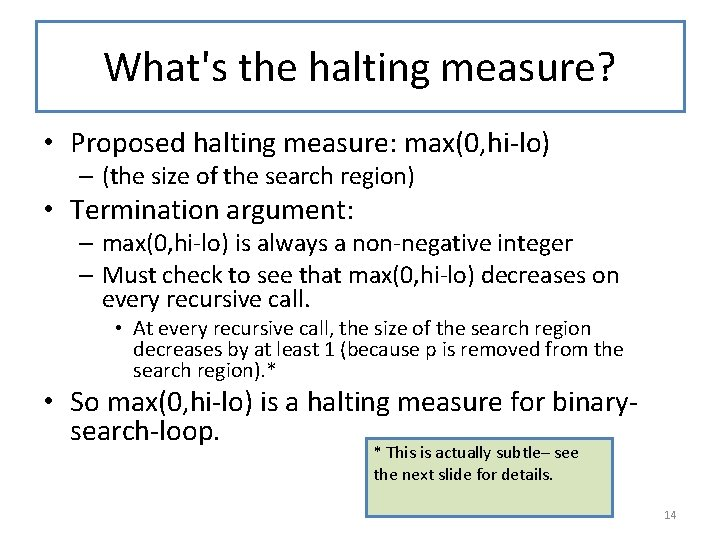What's the halting measure? • Proposed halting measure: max(0, hi-lo) – (the size of the search region) • Termination argument: – max(0, hi-lo) is always a non-negative integer – Must check to see that max(0, hi-lo) decreases on every recursive call. • At every recursive call, the size of the search region decreases by at least 1 (because p is removed from the search region). * • So max(0, hi-lo) is a halting measure for binarysearch-loop. * This is actually subtle– see the next slide for details. 14Checking that the halting measure decreases • Let’s try the first case: – We have • lo < hi • lo ≤ p ≤ hi • f(p) < tgt [that’s how we got to the cond clause] [that’s how we chose p] [that’s the case we are considering. ] – So hi-lo > 0, so max(0, hi-lo) = hi-lo. – In this case we set lo 1 (the new value of lo) to be p+1, and hi 1, the new value of hi, to be equal to hi. – Now we can calculate: hi 1 -lo 1 = hi-(p+1) [substituting values of hi 1 and lo 1] < hi – p [since p < p+1] ≤ hi – lo [since lo ≤ p] – – • So (h 1 -lo 1) < (hi-lo). If hi 1 -lo 1 ≥ 0, then max(0, hi 1 -lo 1) = hi 1 -lo 1 < (hi-lo) = max(0, hi-lo) If hi 1 -lo 1 < 0, then max(0, hi 1 -lo 1) = 0 < hi-lo = max(0, hi-lo) So either way, we have max(0, hi 1 -lo 1) < max(0, hi-lo), and the halting measure has decreased. The other case is similar, of course. Yes, making this argument bullet-proof is tricky. But this merely reflects the fact it’s easy to write sloppy binary search code that will sometimes fail to terminate. So either way you have to be careful. 15Summary • You should now be able to: – explain what binary search is and when it is appropriate – explain how the standard binary search works, and how it fits into the framework of general recursion – give the halting measure and explain the termination argument for binary search – write variations on a binary search function 16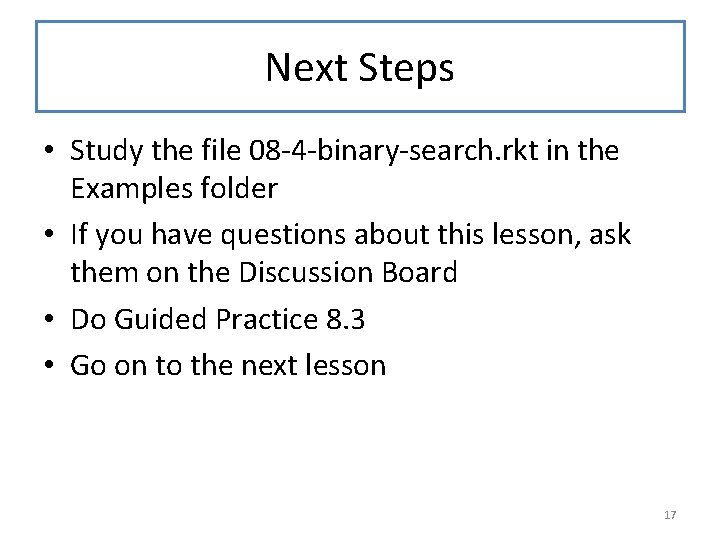Next Steps • Study the file 08 -4 -binary-search. rkt in the Examples folder • If you have questions about this lesson, ask them on the Discussion Board • Do Guided Practice 8. 3 • Go on to the next lesson 17